# 电子罗盘

## 实验介绍

“电子罗盘”，作为一种非常常见的功能，目前市场上的智能手机几乎都默认配备了。在生活中，它可以帮助我们找准方向，进行辅助定位等等。除了手机之外，电子罗盘也广泛应用于航空、航天、机器人、航海、车辆自主导航等领域。在本实验中，我们也来实现一个“电子罗盘”。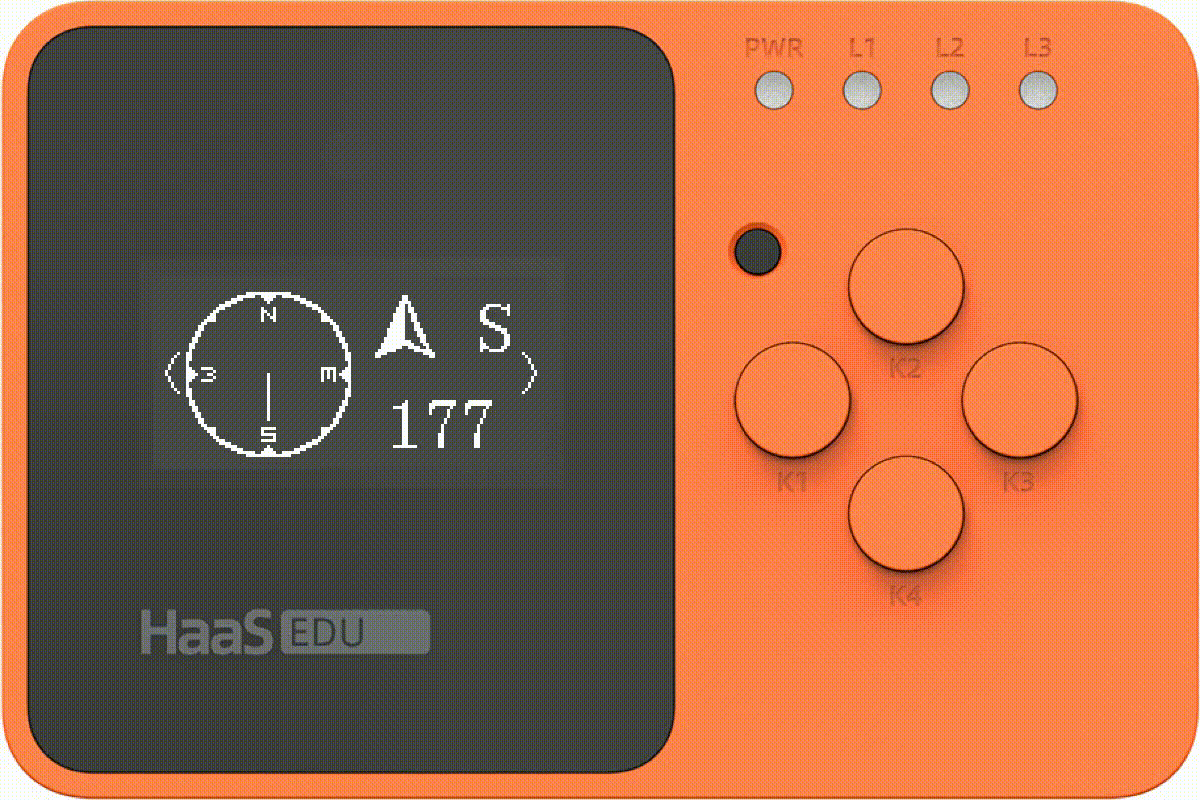## 涉及知识点

• QMC5883L 三轴AMR磁传感器的原理和使用

• OLED绘图

## 硬件

``````开发用电脑一台
HAAS EDU K1 开发板一块
USB2TypeC 数据线一根``````

## 软件

> 选择解决方案: “HaaS EDU K1教育开发案例合集”

> 选择开发板: haaseduk1 board configure

-- 编译固件可参考《AliOS Things集成开发环境使用说明之编译固件》

-- 烧录固件可参考《AliOS Things集成开发环境使用说明之烧录固件》

## 硬件规格

QMC5883L是一款多芯片三轴磁传感器。旨在用于无人机，机器人，移动和个人手持设备中的指南针，导航和游戏等高精度应用。QMC5883L 采用异相磁阻（anisotropic magnetoresistance AMR）技术，这些各向异性传感器具有在轴向高灵敏度和线性高精度的特点。传感器具有的对正交轴低灵敏度的固相结构能用于测量地球磁场的方向和大小，其测量范围从毫高斯到 8 高斯。它具有低噪声，高精度，低功耗，失调消除和温度补偿的优点。 QMC5883L可实现1°至2°的罗盘航向精度。 使用I2C串行总线简化接口。 更详细的硬件规格参数可以参考 DataSheet 的 2.1 Product Specifications

## 传感原理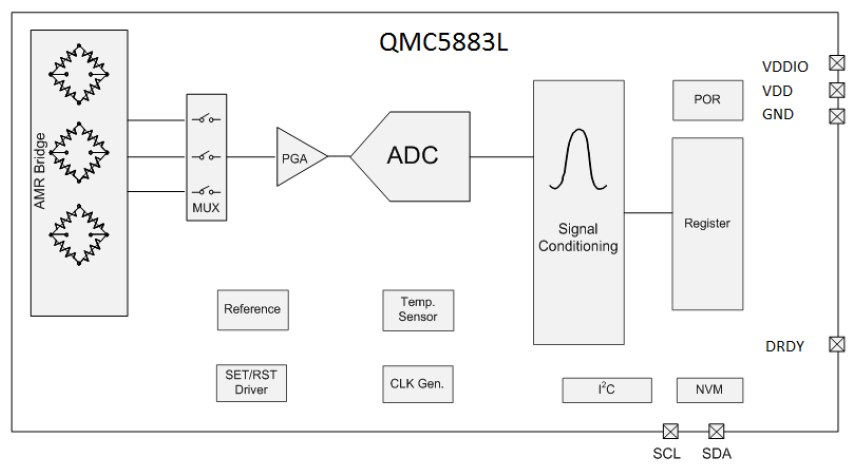• AMR Bridge：三轴异相磁阻传感器，其结构是一个电阻电桥，其电阻会随着所施加的磁场而变化。三个传感器相互正交，从而能够得出三个方向上的磁场强度。

• MUX：多路复用通道，通过开关选中对应的通路，可以采集该通路的传感器数据。

• PGA：可编程控制的传感器信号增益放大器。

• Signal Conditioning：进行磁场信号校正及补偿的数字模块。

• NVM：用于校正的非易失性存储器。

• SET/RST Driver：用于初始化磁性传感器的内部驱动。

• Reference：用于内部偏移的电压/电流基准。

• Temperature Sensor：用于内部精度/偏移的温度传感器，也可以用于测量温度并输出。

## 驱动方式

``````int32_t ret = sensor_i2c_open(QMC5883L_I2C_PORT, QMC5883L_ADDR, I2C_BUS_BIT_RATES_100K, 0);
if (ret) {
LOGE("SENSOR", "sensor i2c open failed, ret:%d\n", ret);
return;
}``````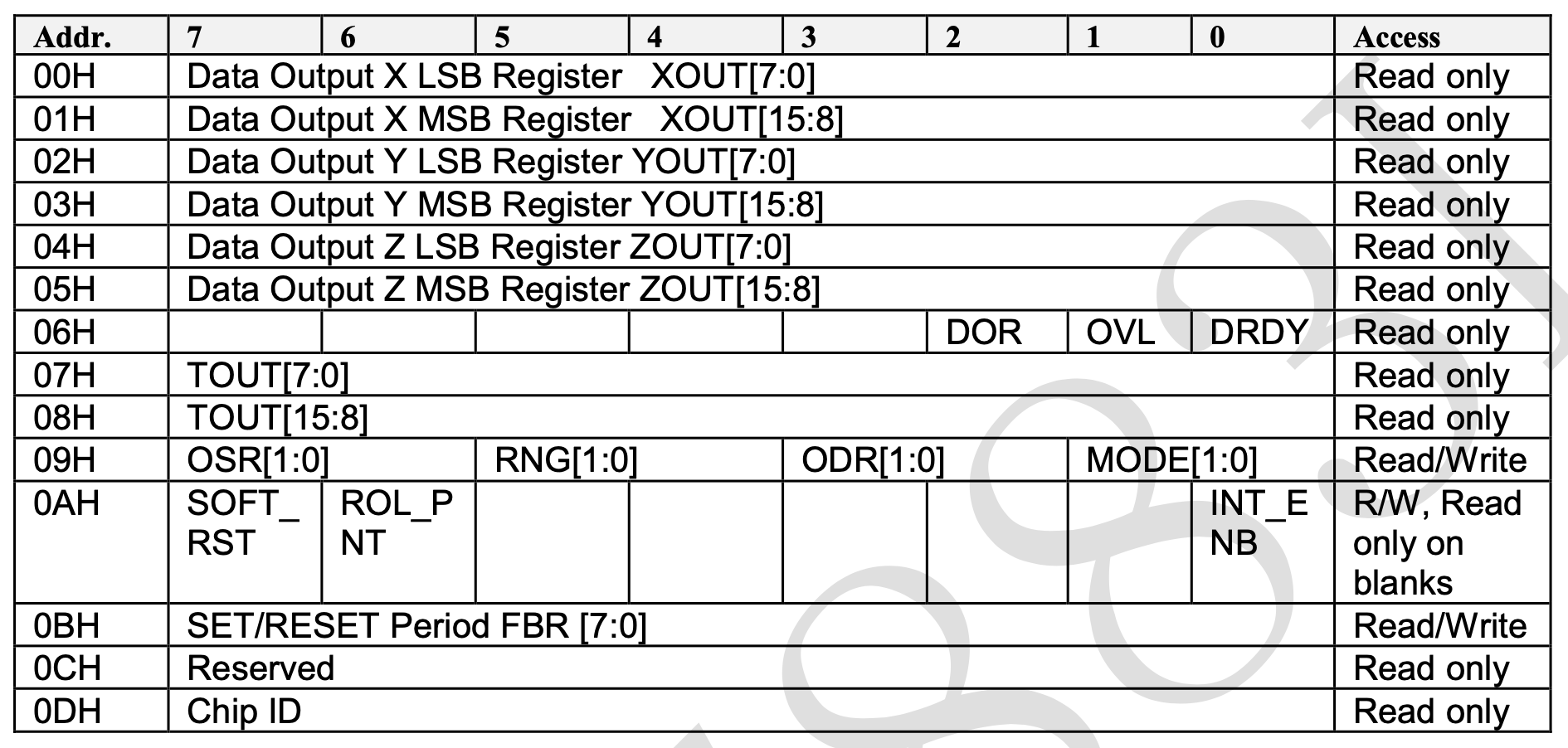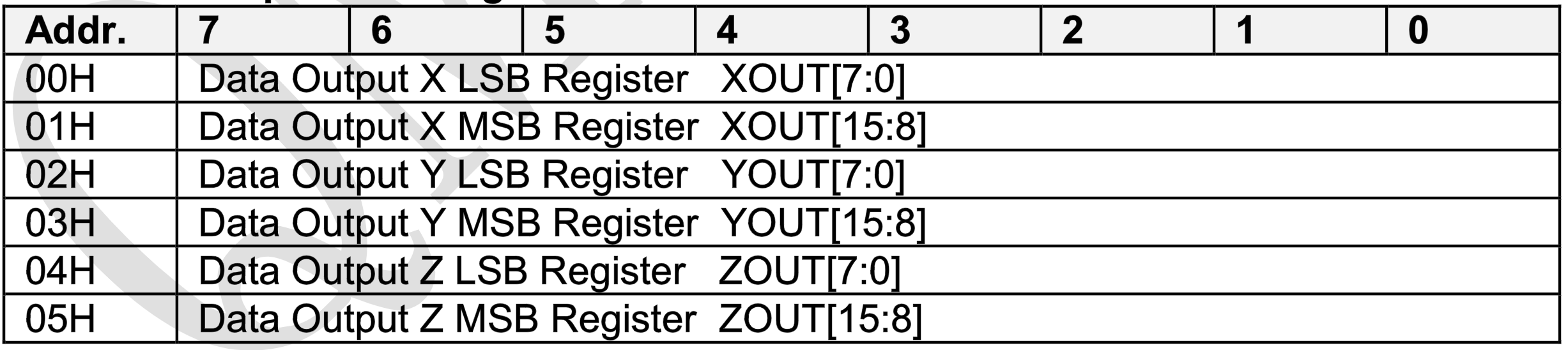• Data Output X Y Z LSB&MSB 00H-05H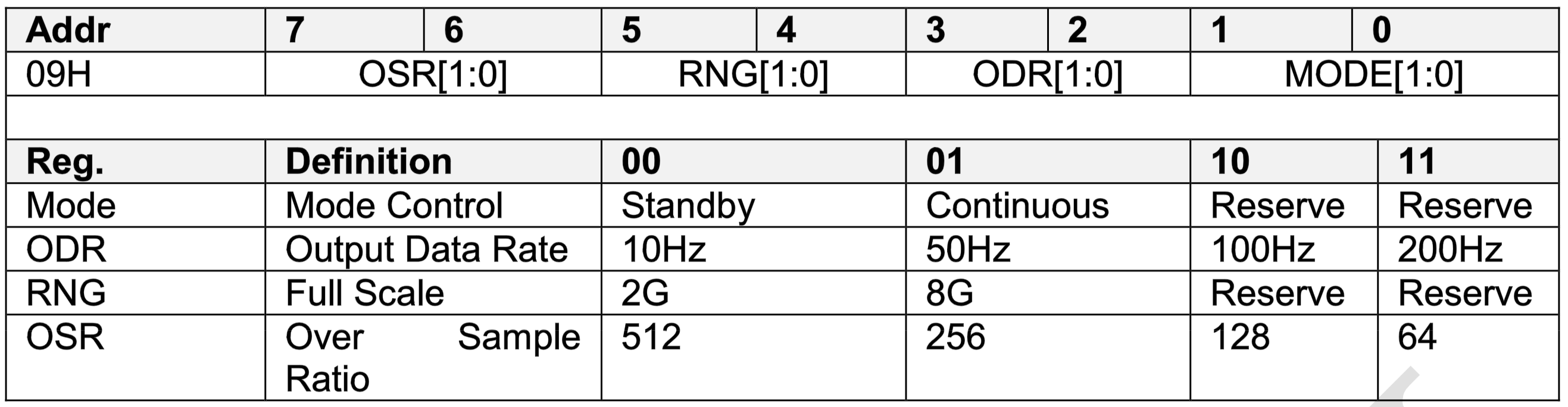• OSR

Over Sample Ratio 过采样率寄存器。用于控制内部数字滤波器的带宽。较大的OSR值会带来较小的滤波器带宽，以及更少的带内噪声和更高的功耗。可以通过配置此寄存器达到噪声和功率之间的良好平衡。

• RNG

Range 量程选择寄存器。可以通过寄存器RNG配置磁传感器的感应范围。量程的选择应该是由具体的应用场景决定的。对于磁性清晰的环境，在弱磁场环境下，例如 +/- 2 gauss 应当选用较小的量程。量程与磁传感器的灵敏度息息相关。在采样位数不变的情况下，最低量程具有最高的灵敏度，因此分辨率更高。

• ODR

Output Data Rate 输出数据频率寄存器。

• MODE

``````// solutions/eduk1_demo/drivers/sensor/drv_mag_honeywell_qmc5883l.c
// solutions/eduk1_demo/drivers/sensor/drv_mag_honeywell_qmc5883l.h

static void _qmc5883l_init() {
/* This assumes the wire library has been initialized. */
oversampling = QMC5883L_CONFIG_OS512;                // 512倍过采样
range = QMC5883L_CONFIG_8GAUSS;                                // 8高斯量程
rate = QMC5883L_CONFIG_200HZ;                                // 200Hz数据输出频率
mode = QMC5883L_CONFIG_CONT;                                // 开启连续采样模式
qmc5883l_reset();
}``````

``````// solutions/eduk1_demo/drivers/sensor/drv_mag_honeywell_qmc5883l.c
// solutions/eduk1_demo/drivers/sensor/drv_mag_honeywell_qmc5883l.h

# define QMC5883L_CONFIG2 0x09
# define QMC5883L_CONFIG2 0x0a

static qmc5883l_write_register(uint8_t addr, uint8_t reg, uint8_t data)
{
uint8_t write_buffer = {reg, data};
qmc5883l_i2c_master_send(write_buffer, 2, 1000);
}

static void qmc5883l_reconfig()
{
}``````

``````// solutions/eduk1_demo/drivers/sensor/drv_mag_honeywell_qmc5883l.c
// solutions/eduk1_demo/drivers/sensor/drv_mag_honeywell_qmc5883l.h

{
qmc5883l_i2c_master_send(&reg, 1, 1000);
aos_msleep(20);
qmc5883l_i2c_master_recv(buf, len, 1000);
return 1;
}

int qmc5883l_readRaw(int16_t *x, int16_t *y, int16_t *z)
{
uint8_t data;
uint32_t timeout = 10000;

*x = data | (data<<8);
*y = data | (data<<8);
*z = data | (data<<8);

printf("get org data [%d,%d,%d]\n", x, y, z);

return 1;
}``````

``````atan2(x_fit, y_fit) * 180.0 / M_PI;
// atan2(x, y) 返回以弧度表示的 y/x 的反正切``````

## 数据校准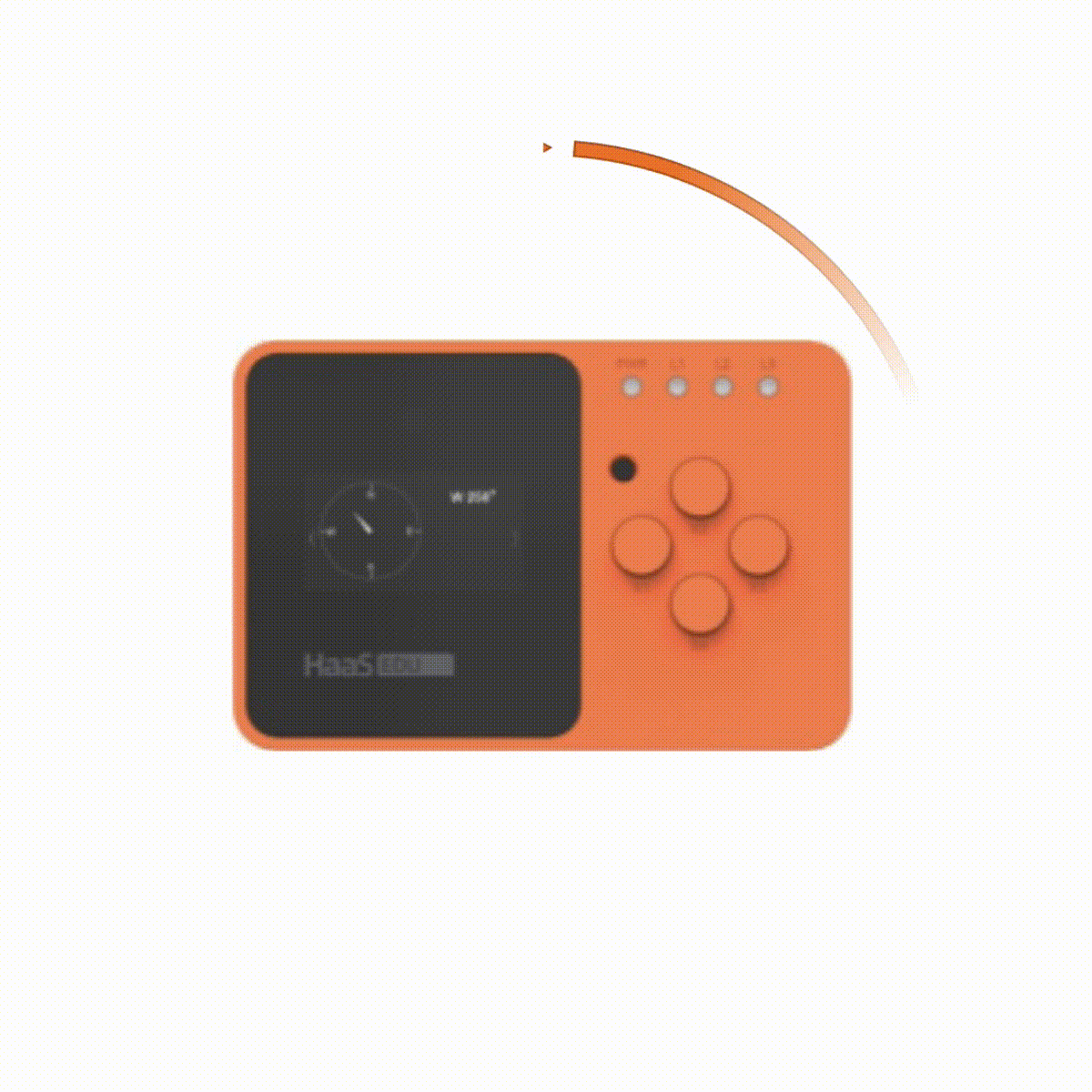``````org_list = [
[-1438,-1690,-2665],
[-1438,-1690,-2665],
[-1446,-1660,-2667],
[-1418,-1642,-2702],
...
]``````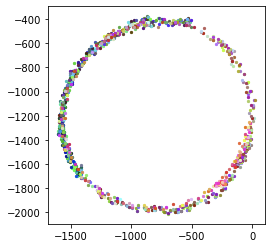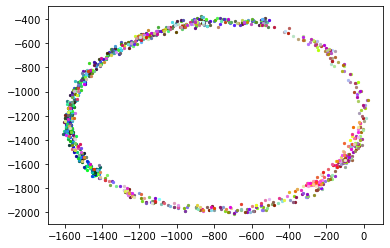``\dfrac{x-x_{offset}}{x_{\max}-x_{\min }}=\dfrac{y-y_{offset}}{y_{\max}-y_{\min }}=\dfrac{z-z_{offset}}{z_{\max}-z_{\min }}``

`````` x_max = y_max = z_max = INT16_MIN;
x_min = y_min = z_min = INT16_MAX;

{
int16_t x_org, y_org, z_org;                // 原始数据
float x_offset, y_offset, z_offset;        // 球心坐标偏移
float x_fit, y_fit, z_fit;                        // 修正数据

return 0;

// 在每次读取数据时都更新数据边界
x_min = x_org < x_min ? x_org : x_min;
x_max = x_org > x_max ? x_org : x_max;
y_min = y_org < y_min ? y_org : y_min;
y_max = y_org > y_max ? y_org : y_max;
z_min = z_org < z_min ? z_org : z_min;
z_max = z_org > z_max ? z_org : z_max;

// 如果数据量不足以计算边界 则退出
if (x_min == x_max || y_min == y_max || z_max == z_min)
return 0;

// 通过边界计算出圆心偏移
x_offset = (x_max + x_min) / 2.0;
y_offset = (y_max + y_min) / 2.0;
z_offset = (z_max + z_min) / 2.0;

// 修正比例
x_fit = (x_org - x_offset) * 1000.0 / (x_max - x_min);
y_fit = (y_org - y_offset) * 1000.0 / (y_max - y_min);
z_fit = (z_org - z_offset) * 1000.0 / (z_max - z_min);

printf("fix[%f,%f,%f],\n", x_fit, y_fit, z_fit);

int heading = 180.0 * atan2(x_fit, y_fit) / M_PI;

}``````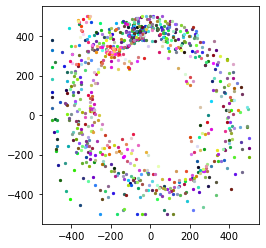## 界面开发

``````while (1)
{